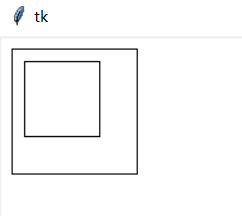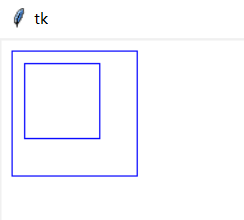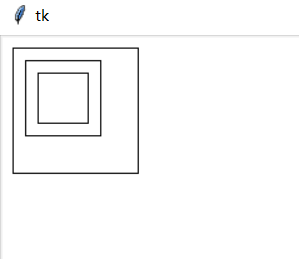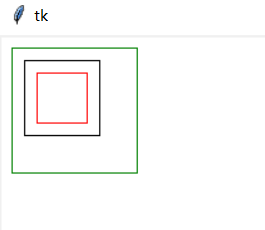## tkinter -- Canvas(2)

创建 item 的 tags

使用属性 tags 设置 item 的 tag

使用 Canvas 的方法 gettags 获取指定 item 的 tags

代码:

```import tkinter as tk
root = tk.Tk()
# 创建一个 Canvas，设置其背景色为白色
cv = tk.Canvas(root, bg='white')

# 使用 tags 指定一个 tags('r1')
rt = cv.create_rectangle(10, 10, 110, 110, tags='r1')
cv.pack()
print(cv.gettags(rt))
# 使用 tags 属性指定多个 tags,即重新设置 tags 的属性
cv.itemconfig(rt, tags=('r2', 'r3', 'r4'))
print(cv.gettags(rt))
root.mainloop()```

结果:多个 item 使用同一个 tag

多个控件使用同一个 tag

代码:

```import tkinter as tk
root = tk.Tk()
# 创建一个 Canvas，设置其背景色为白色
cv = tk.Canvas(root, bg='white')

# 使用 tags 指定一个 tags('r1')
rt = cv.create_rectangle(10, 10, 110, 110, tags=('r1', 'r2', 'r3'))
cv.pack()
cv.create_rectangle(20,20,80,80,tags='r3')
print(cv.find_withtag('r3'))
root.mainloop()```

结果:动态修改 item 的坐标

find_withtag 返回所有与 tag 绑定的 item

通过 tag 来访问 item

得到了 tag 值也就得到了这个 item，可以对这个 item 进行相关的设置

代码:

```import tkinter as tk
root = tk.Tk()
# 创建一个 Canvas，设置其背景色为白色
cv = tk.Canvas(root, bg='white')

# 使用 tags 指定一个 tags('r1')
rt = cv.create_rectangle(10, 10, 110, 110, tags=('r1', 'r2', 'r3'))
cv.pack()
cv.create_rectangle(20, 20, 80, 80,tags='r3')
# 将所有与tag('r3')绑定的item边框颜色设置为蓝色
for i in cv.find_withtag('r3'):
cv.itemconfig(i, outline='blue')
root.mainloop()```

结果:动态修改与 tag('r3')绑定的 item 边框颜色

向其它 item 添加 tag

代码:

```import tkinter as tk
root = tk.Tk()
# 创建一个 Canvas，设置其背景色为白色
cv = tk.Canvas(root, bg='white')

# 创建三个rectangle
rt1 = cv.create_rectangle(10, 10, 110, 110, tags=('r1', 'r2', 'r3'))
rt2 = cv.create_rectangle(20, 20, 80, 80, tags=('s1', 's2', 's3'))
rt3 = cv.create_rectangle(30, 30, 70, 70, tags=('y1', 'y2', 'y3'))
# 向 rt2的上一个 item 添加 r4
# 向 rt2的下一个 item 添加 r5

for i in [rt1, rt2, rt3]:
print(cv.gettags(i))
cv.pack()
root.mainloop()```

结果:Canvas 使用了 stack 的技术，新创建的 item 总是位于前一个创建的 item 之上，故调用above 时，它会查找 rt2上面的 item 为 rt3,故 rt3中添加了 tag('r4')，同样 add_below 会查找下面的 item

返回其它 item

使用 find_xxx 查找上一个或下一个 item

代码:

```import tkinter as tk
root = tk.Tk()
# 创建一个 Canvas，设置其背景色为白色
cv = tk.Canvas(root, bg='white')

# 创建三个rectangle
rt1 = cv.create_rectangle(10, 10, 110, 110, tags=('r1', 'r2', 'r3'))
rt2 = cv.create_rectangle(20, 20, 80, 80, tags=('s1', 's2', 's3'))
rt3 = cv.create_rectangle(30, 30, 70, 70, tags=('y1', 'y2', 'y3'))
# 查找 rt2的上一个 item,并将其边框颜色设置为红色
cv.itemconfig(cv.find_above(rt2), outline='red')
# 查找 rt2的下一个 item,并将其边框颜色设置为绿色
cv.itemconfig(cv.find_below(rt2), outline='green')

cv.pack()
root.mainloop()```

结果:Canvas 使用了 stack 的技术，新创建的 item 总是位于前一个创建的 item 之上，故调用above 时，它会查找 rt2上面的 item 为 rt3,故 rt3中边框颜色设置为红色，同样 add_below会查找下面的 item